﻿ 可破碎颗粒体在动力载荷下的耗能特性
«上一篇文章快速检索 高级检索

 力学学报2015, Vol. 47Issue (2): 252-259  DOI: 10.6052/0459-1879-14-1450

### 引用本文 [复制中英文]

[复制中文]
Qi Yuan, Huang Junjie, Chen Mingxiang. ENERGY DISSIPATION CHARACTERISTICS OF CRUSHABLE GRANULES UNDER DYNAMIC EXCITATIONS[J]. Chinese Journal of Ship Research, 2015, 47(2): 252-259. DOI: 10.6052/0459-1879-14-145.
[复制英文]

### 文章历史

2014-05-23 收稿
2014-07-12 录用
2014–09-17 网络版发表

1 基本原理

1.1 颗粒体连接键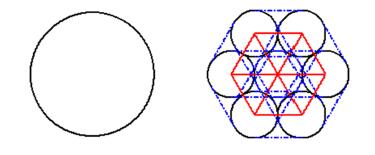图 1 颗粒体代替单个颗粒示意图(红线表示接触键,蓝线表示平行键) Fig. 1 Schematic picture of the repalcement of single particle by cluster.(Red line stands for contact bond, and blue line stands for paralleled bond)

1.2 破碎率

 ${B_{\rm{r}}} = \frac{{{N_{{\rm{br}}}}}}{{{N_{{\rm{ini}}}}}}$ (1)

1.3 能量

 ${E_{\rm{b}}} = \sum\limits_{t = 0}^t {\sum\limits_{p = 1}^{{N_p}} {\rm{ }} } {m_p}g{\rm{d }}{u_p}{\rm{d }}t$ (2)

 ${E_w} = \sum\limits_{t = 0}^t {\sum\limits_{w = 1}^{{N_w}} {\left( {{f_w}{\rm{d}}{u_w} + {t_w}{\rm{d}}{\theta _w}} \right)} } {\rm{ d}}t$ (3)

 ${E_k} = \frac{1}{2}\sum\limits_{p = 1}^{{N_p}} {\left( {{m_p}\dot u_p^2 + {I_p}\omega _p^2} \right)}$ (4)

 ${E_{\rm{s}}} = \frac{1}{2}\sum\limits_{c = 1}^{{N_{\rm{c}}}} {\left( {\frac{{f_{\rm{n}}^2}}{{{k_{\rm{n}}}}} + \frac{{f_{\rm{t}}^2}}{{{k_{\rm{t}}}}}} \right)}$ (5)

 ${E_{\rm{f}}} = \sum\limits_{t = 0}^t {\sum\limits_{c = 1}^{{N_{\rm{c}}}} {{f_{\rm{t}}}d{u_{{\rm{slip}}}}{\rm{d}}} } t$ (6)

 ${E_{\rm{d}}} = \sum\limits_{t = 0}^t {\sum\limits_{c = 1}^{{N_{\rm{c}}}} {\left[{\left( {{c_{\rm{n}}}{{\mathop u\limits^. }_{\rm{n}}}} \right){\rm{d}}{u_{\rm{n}}} + \left( {{c_{\rm{t}}}{{\mathop u\limits^. }_{\rm{t}}}} \right){\rm{d}}{u_{\rm{t}}}} \right]} } {\rm{d}}t$ (7)

 ${E_{{\rm{pb}}}} = \frac{1}{2}\sum\limits_{c = 1}^{{N_{{\rm{pb}}}}} {\left( {\frac{{f_{{\rm{pbn}}}^2}}{{{A_{{\rm{pb}}}}{k_{{\rm{pbn}}}}}} + \frac{{f_{{\rm{pbt}}}^2}}{{{A_{{\rm{pb}}}}{k_{{\rm{pbt}}}}}} + \frac{{t_{{\rm{pb}}}^2}}{{{I_{{\rm{pb}}}}{k_{{\rm{pbn}}}}}}} \right)}$ (8)

 ${E_{{\rm{br}}}} = \sum\limits_{{c_{{\rm{br}}}} = 1}^{{c_{{\rm{br}}}}} {E_{{\rm{bp}}}^{\max }{\rm{d}}{c_{{\rm{br}}}}}$ (9)

 ${E_w} = {E_{\rm{b}}} + {E_k} + {E_{\rm{s}}} + {E_{\rm{f}}} + {E_{\rm{d}}} + {E_{{\rm{pb}}}} + {E_{{\rm{br}}}}$ (10)
2 数值颗粒微观参数及动力载荷参数选取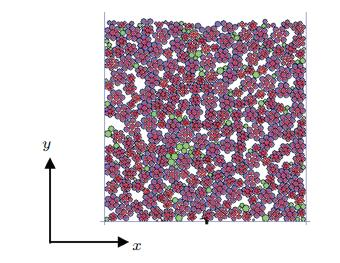图 2 样本示意图 Fig. 2 2D computational model

 $\left. \begin{array}{l} {{\dot u}_x}\left( t \right) = \frac{{{v_p}}}{2}\sin \left( {{\alpha _p}t} \right),0 \le t \le T\\ {{\dot u}_y}\left( t \right) = {v_p}\sin \left( {{\alpha _p}t + \pi } \right),0 \le t \le T \end{array} \right\}$ (11)

3 结果和讨论

3.1 颗粒体破碎及系统耗能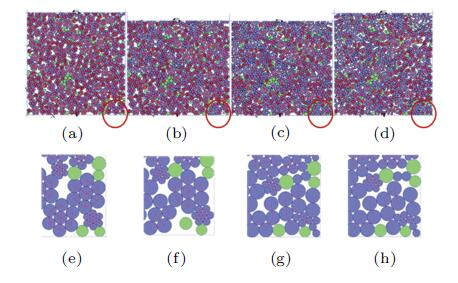图 3 颗粒体破碎情况随时间变化示意图: 由(a)到(d)加载时间分别为0,1/4,3/4,1个周期, (e)到(h)为相应红圈区域放大图(颗粒间有效连接键由红线表示) Fig. 3 The progress of cluster breakage: the loading time of the snippets from (a) to (d) are 0,1/4,3/4,1 period, respectively; and the snippets from (e) to (f) are the zooming pictures of the area circled with red lines, vskip -1mm respectively (The red lines indicate the effective contact bonds)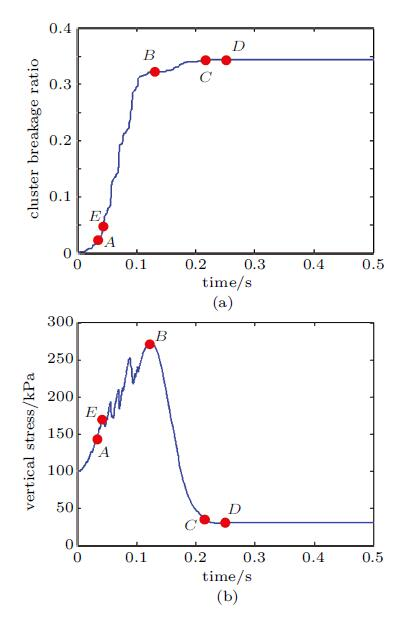图 4 系统破碎率(a) 与竖向应力分量(b) 随时间变化曲线 Fig. 4 The cluster breakage ratio (a) and the vertical stress component (b) time histories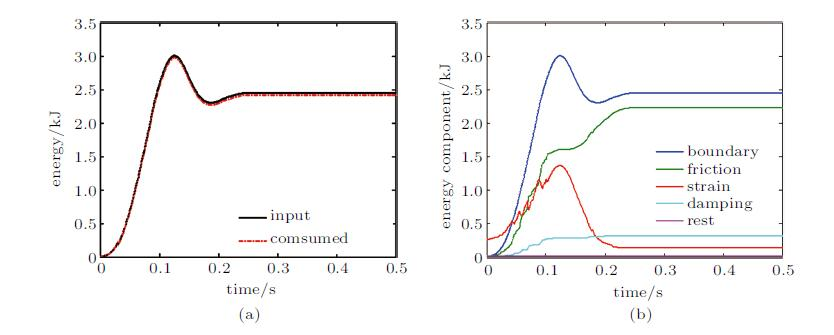图 5 外界输入能量(a) 以及内部消耗能量(b) 随时间变化示意图 Fig. 5 Input (a) and consumed (b) energy time histories
3.2 破碎对系统耗能的影响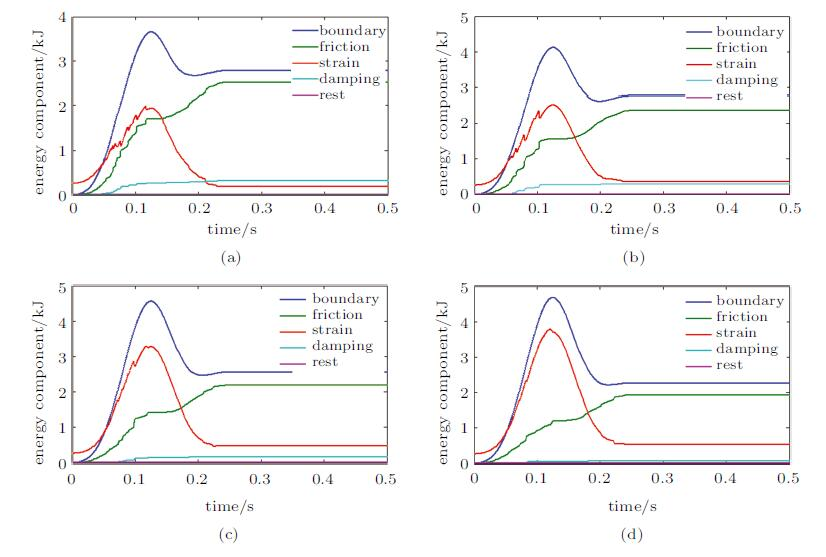图 6 不同连接键强度下系统能量各组成部分随时间的变化(由(a) 到(d) 连接键强度分别为1.5, 2.0, 3.0, 5.0 kN) Fig. 6 Energy components time histories of different contact bond values (The bond strengths from (a) to (d) are 1.5, 2.0, 3.0, 5.0 kN, respectively)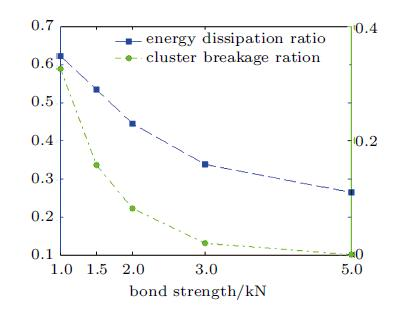图 7 外界累计最大输入能量时连接键强度对能量耗散率和破碎率的影响 Fig. 7 The influence of contact bond value on energy dissipation ratio and cluster breakage ratio
3.3 循环载荷下破碎率和系统耗能的变化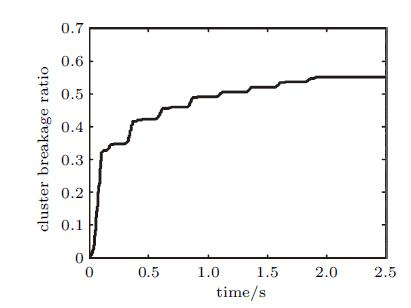图 8 循环载荷下破碎率随时间的变化 Fig. 8 Cluster breakage ratio time history under cyclic loading
4 结 论

  Deluzarche R, Cambou B. Discrete numerical modelling of rockfill dams. International Journal for Numerical and Analytical Methods in Geomechanics, 2006, 30(11): 1075-1096  石云, 郑月秀. 大粒径填石路堤碾压试验研究. 公路交通技术, 2011, 6: 13-15 (Shi Yun, Zheng Yuexiu. Experimental research on rolling compaction of large particle size rock filling roadbed. Technology of Highway and Transport, 2011, 6: 13-15 (in Chinese))  Bai XM, Keer L, Wang QJ, et al. Investigation of particle damping mechanism via particle dynamics simulations. Granular Matter, 2009, 11(6): 417-429  Bagi K. Stress and strain in granular assemblies. Mechanics of Materials, 1996, 22(3): 165-177  Li X, Yu HS, Li XS. Macro-micro relations in granular mechanics. International Journal of Solids and Structures, 2009, 46(25-26): 4331-4341  Li X, Li XS. Micro-macro quantification of the internal structure of granular materials. Journal of Engineering Mechanics, 2009, 135(7): 641-656  Millet O, Gu S, Kondo D. A 4th order fabric tensor approach applied to granular media. Computers and Geotechnics, 2009, 36(5): 736-742  Sitharam TG. Discrete element modelling of cyclic behaviour of granular materials. Geotechnical & Geological Engineering, 2003, 21(4): 297-329  Ng T, Dobry R. Numerical simulations of monotonic and cyclic loading of granular soil. Journal of Geotechnical Engineering, 1994, 120(2): 388-403  孔宪京, 刘君, 韩国城. 面板堆石坝模型动力破坏试验与数值仿真分析. 岩土工程学报, 2003, 25(1): 26-30 (Kong Xianjing, Liu Jun, Han Guocheng. Dynamic failure test and numerical simulation of model concrete-faced rockfill dam. Chinese J. Geot. Eng., 2003, 25(1): 26-30 (in Chinese))  杨庆华, 姚令侃, 杨明. 地震作用下松散堆积体崩塌的颗粒流数值模拟. 西南交通大学学报, 2009, 44(4): 580-584 (Yang Qinghua, Yao Lingkan, Yang Ming. Particle flow numerical simulation of landslip of loose slope under seismic loading. Journal of Southwest Jiaotong University, 2009, 44(4): 580-584 (in Chinese))  Huang JJ, Qi Y, Chen MX, Micromechanical response of granular geomaterials subjected to earthquake ground motions. In: Proc. of International Conference on Science and Technology of Heterogeneous Materials and Structures, Wuhan. 2013.  Chen T, Mao K, Huang X, et al., Dissipation mechanisms of nonobstructive particle damping using discrete element method. Smart Structures and Materials: Damping and Isolation, 2001.4331: 294-301.  黄俊宇, 徐松林, 胡时胜. 脆性颗粒材料的应变率效应机理研究. 固体力学学报, 2013, 34(3): 247-250 (Huang Junyu, Xu Songlin, Hu Shisheng. Inverstifation on the intrinsic mechanisms of strain rate effects of brittle granular materials. Chinese Journal of Solid Mechanics, 2013, 34(3): 247-250 (in Chinese))  刘君, 刘福海, 孔宪京. 考虑破碎的堆石料颗粒流数值模拟. 岩土力学, 2008, 29(增1): 107-112 (Liu Jun, Liu Fuhai, Kong Xianjing. Particle flow code numerical simulation of particle breakage of rockfill. Rock and Soil Mechanics, 2008, 29(sup.1): 107-112 (in Chinese))  马刚, 周伟, 常晓林, 等. 考虑颗粒破碎的堆石体三维随机多面体细观数值模拟. 岩石力学与工程学报, 2011, 30(8): 1671-1682 (Ma Gang, Zhou Wei, Chang Xiaolin, et al. Mesoscopic numeiacal simulation of rockfill considering particle breakage by using three-dimensional stochatic polyhedrons. Chinese Journal of Rock Mechancs and Engineering, 2011, 30(8): 1671-1682 (in Chinese))  Huang J, Xu S, Hu S. Effects of grain size and gradation on the dynamic responses of quartz sands. International Journal of Impact Engineering, 2013, 59: 1-10  Huang JY, Xu SL, Hu SS. Influence of particle breakage on the dynamic compression responses of brittle granular materials. Mechanics of Materials, 2014, 68: 15-28  刘汉龙, 孙逸飞, 杨贵, 等. 粗粒料颗粒破碎特性研究述评. 河海大学学报(自然科学版), 2012, 40(4): 361-369 (Liu Hanlong, Sun Yifei, Yang Gui, et al. A review of particle breakage characteristics of coarse aggregates. Journal of Hohai University (Natural Sciencesm ), 2012, 40(4): 361-369 (in Chinese))  Cundall PA, Strack ODL, A discrete numerical model for granular assemblies. G Technique, 1979, 29: 47-65.  Cho N, Martin CD, Sego DC. A clumped particle model for rock. International Journal of Rock Mechanics and Mining Sciences, 2007, 44(7): 997-1010  Potyondy DO, Cundall PA. A bonded-particle model for rock. International Journal of Rock Mechanics and Mining Sciences, 2004, 41(8): 1329-1364  Jensen R, Edil T, Bosscher P, et al. Effect of particle shape on interface behavior of DEM —— simulated granular materials. International Journal of Geomechanics, 2001, 1(1): 1-19  Jensen R, Plesha M, Edil T, et al. DEM simulation of particle damage in granular media —— structure interfaces. International Journal of Geomechanics, 2001, 1(1): 21-39  El Shamy U, Denissen C. Microscale characterization of energy dissipation mechanisms in liquefiable granular soils. Computers and Geotechnics, 2010, 37(7-8): 846-857  黄俊宇, 徐松林, 王道荣, 等. 脆性颗粒材料的动态多尺度模型研究. 岩土力学, 2013, 34(4): 922-932 (Huang Junyu, Xu Songlin, Wang Daorong, et al. Investigation of dynamic multiscale model for brittle granular materials. Rock and Soil Mechanics, 2013, 34(4): 922-932 (in Chinese))  Lackenby J, Indraratna B, McDowell G, et al. Effect of confining pressure on ballast degradation and deformation under cyclic triaxial loading. G Technique, 2007, 57: 527-536
ENERGY DISSIPATION CHARACTERISTICS OF CRUSHABLE GRANULES UNDER DYNAMIC EXCITATIONS
Qi Yuan, Huang Junjie, Chen MingxiangDepartment of Mechanical Engineering, Wuhan University, Wuhan 430072, China
Fund: This project was supported by The Hubei Provincial Key Laboratory of Safety for Structural and Geotechnical Engineering (HBKLCIV201207), the Young Faculty Research Grant atWuhan University (2042014KF0007), and The National Key Basic Research Development Program (973 Program) sub-project (2014CB046902).
Abstract: Using the discrete element method (DEM) with cluster, different degrees of particle crushing by setting various contact bond thresholds under external dynamic excitation are implemented, and their energy dissipation characteristics are also discussed. Numerical results indicate that the particle breakage ratio has a great influence on the energy dissipation ratio which is defined as consumed energy to the input energy. As the particle breakage ratio rises, which intensify the friction and the collision between particles, the energy dissipation ratio increases. Besides, most cluster disintegration happens on the early stage of cyclic loading. Gradually, the breakage rate slows down, and the energy dissipation ratio reduces.
Key words: discrete element method    particle breakage    breakage ratio    energy dissipation